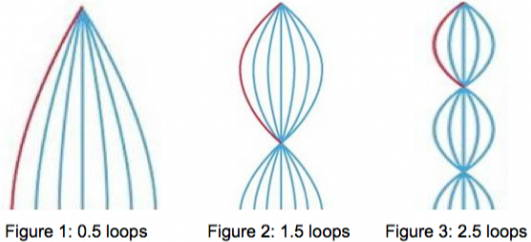Standing Waves Like You've Never Seen Them Before

A standing wave is formed when two identical traveling waves continually pass through the same medium in opposite directions. Not only can standing waves be produced on springs by sending continuous traveling waves down a horizontal spring that is held firmly at the far end, they can also be produced using a spring that is held vertically such that its lower end is free. This results in a node at the upper end of the spring and an antinode at the lower end of the spring, and yields standing waves having 0.5 loops, 1.5 loops, 2.5 loops, 3.5 loops, etc…, as depicted in Figure 1.In the video clip, you will see Arbor Scientific's new Spring Wave (Product #P7-7220) held above the floor.  By adjusting the frequency of the waves, the wavelength may be manipulated so that different numbers of loops can be formed. As with all standing waves, the length of one loop is one-half wavelength.

You can determine the frequency of each standing wave by timing the motion for 10 complete cycles.  The frequency in Hertz will be 10 cycles divided by the time in seconds taken to produce the 10 cycles.  Since the tension in the vertically held spring remains constant for all trials, the speed of the standing waves will be the same, regardless of the number of loops formed.  This allows us to determine the ratio of frequencies needed to produce different standing wave patterns.

Assume we use a spring of length L when suspended vertically.  The standing wave form in Figure 1 having 0.5 loops will have a wave speed v = f0.5λ0.5, where λ0.5 = 4L and f0.5 is the timed frequency.  Therefore, f0.5 = v/4L = ¼ v/L.  Because the tension in the vertical spring has not changed, the standing wave in Figure 2 will have the same speed as the standing wave in Figure 1.  In this case, the wave speed v = f1.5λ1.5, where λ1.5 = 4/3 L = 4L/3.  Therefore, f1.5 = v/4L/3 = 3v/4L = 3(¼ v/L) = 3f0.5.  We should therefore expect that the frequency to produce 1.5 loops will be three times the frequency needed to produce 0.5 loops.  Similarly, the standing wave in Figure 3 will have a wave speed v = f2.5λ2.5, where λ2.5 = 4/5 L = 4L/5.  Therefore, f2.5 = v/4L/5 = 5v/4L = 5(¼ v/L) = 5f0.5.  Comparing f2.5 and f1.5:  f2.5/f1.5 = 5f0.5/3f0.5 = 5/3.  We would therefore expect that the frequency needed to produce 2.5 loops would be 5 times the frequency need to produce 0.5 loops, and 1.67 times the frequency needed to produce 1.5 loops.

In the video, the total time needed to produce 10 cycles when 0.5 loops were formed in the Spring wave was approximately 18.21 sec.  This results in a frequency f of 10 cycles/18.21 sec = 0.549 Hz.  Similarly, the frequency when 1.5 loops were formed = 10 cycles/6.06 s = 1.650 Hz.  The ratio of the frequency to produce 1.5 loops to the frequency to produce 0.5 loops = 1.650/0.549 = 3.01, which is quite close to the expected ratio of 3.00.

For 2.5 loops, the frequency is found to be 2.882 Hz (10 cycles in 3.47 seconds).  This frequency is approximately 5.25 times as great as the frequency to produce 0.5 loops, which is close to the expected value of 5.00, and 1.75 times as great as the frequency to produce 1.5 loops.

Slight discrepancies in the calculated ratios can likely be attributed to errors in measuring time and quite likely in not producing the most "perfect" standing wave form.  The actual ratios your students measure when they perform this investigation for themselves will depend on the types and lengths of springs used.

The video clip concludes with vertical standing waves produced using another plastic toy spring.  Although the measured frequencies will differ using springs with different lengths, diameters, and elastic constants, the ratios of frequencies needed to produce the standing waves should be the same.

See Joel's other wave demo: Big Standing Wave – Small Effort!

Collin Wassilak